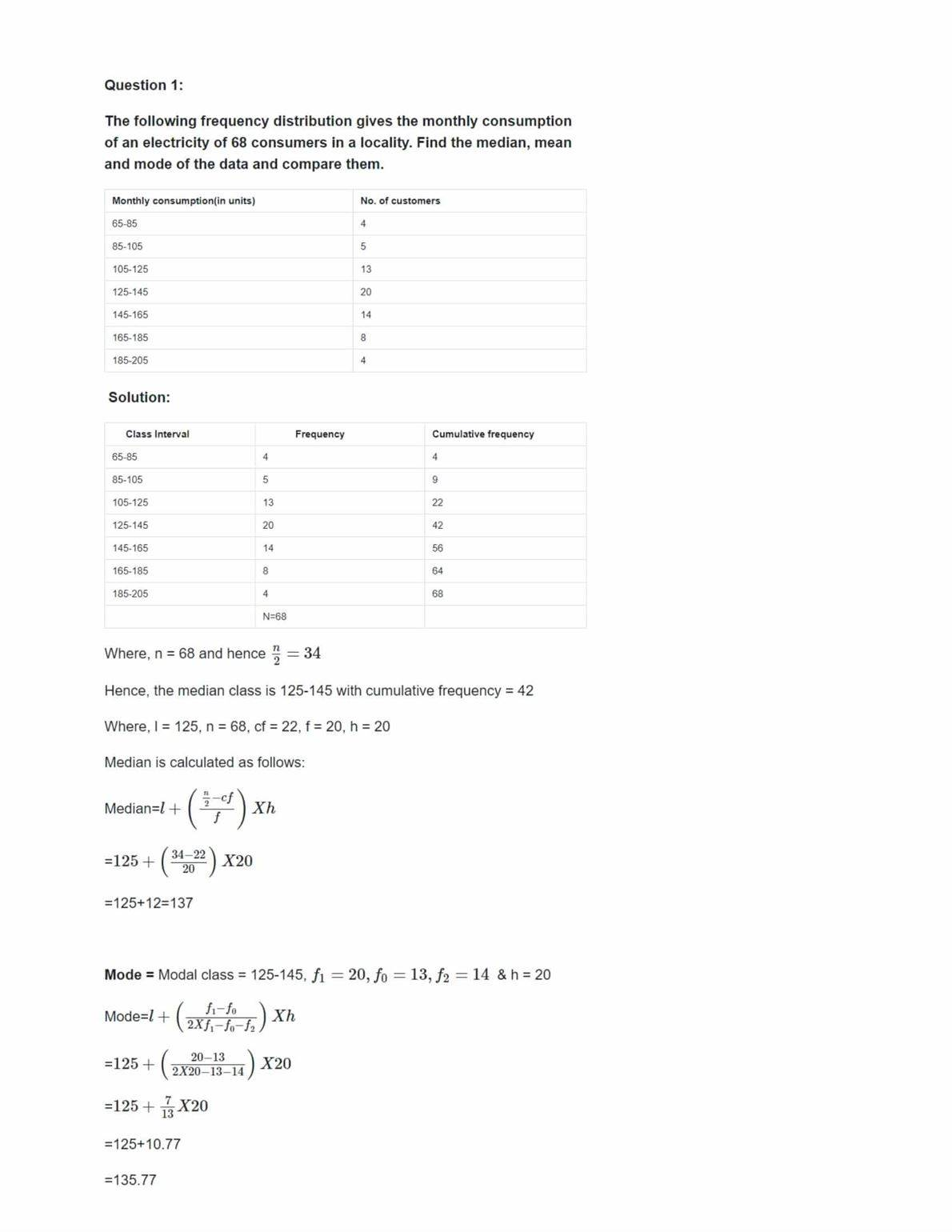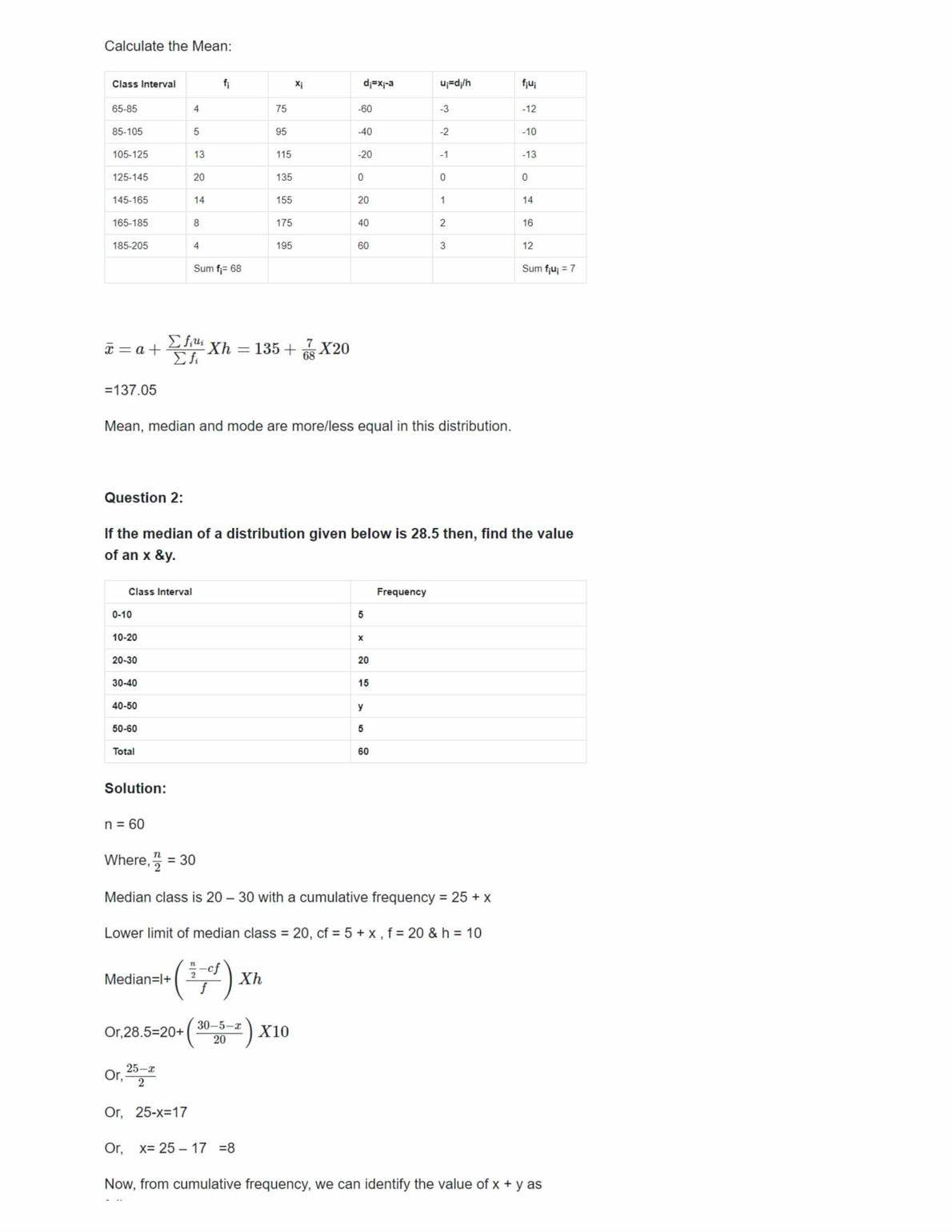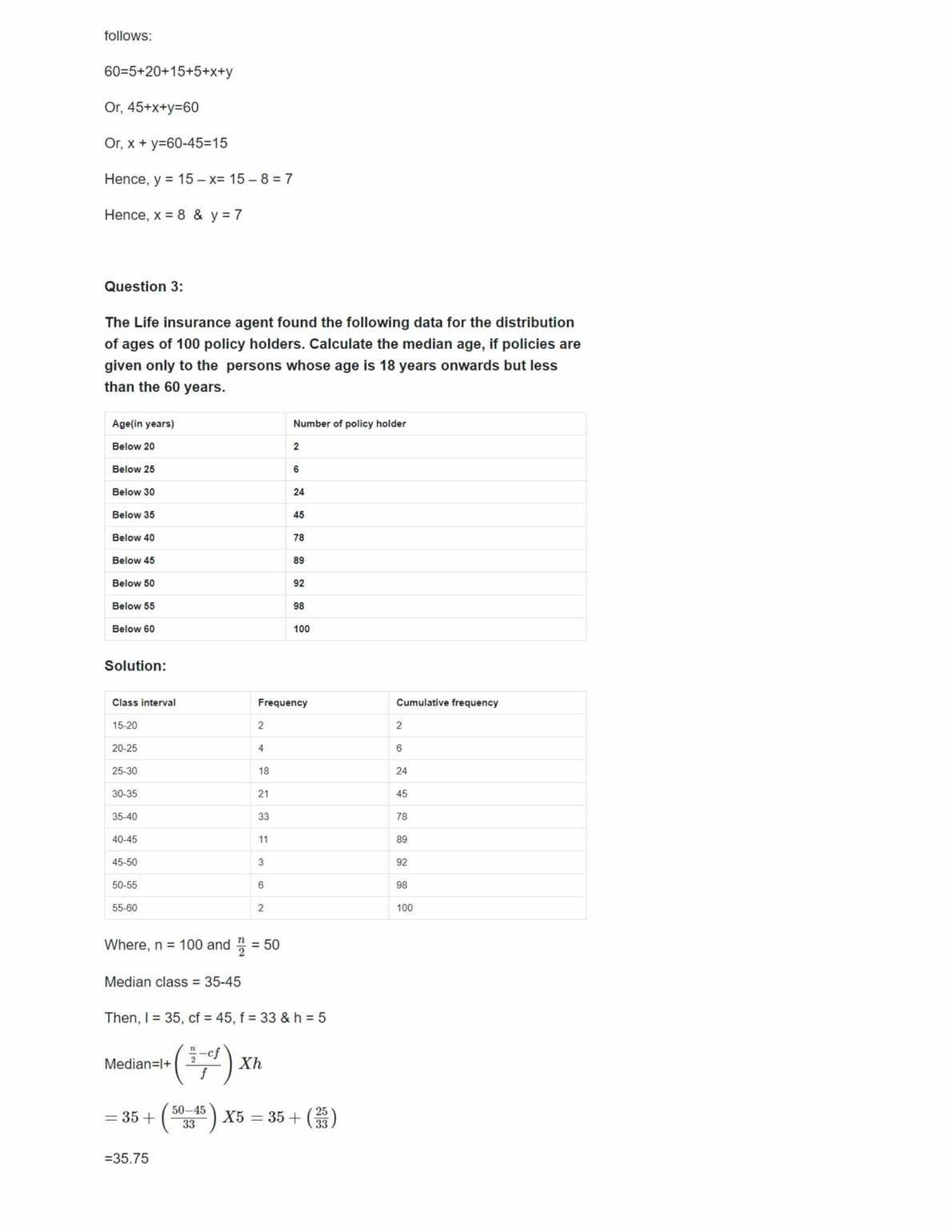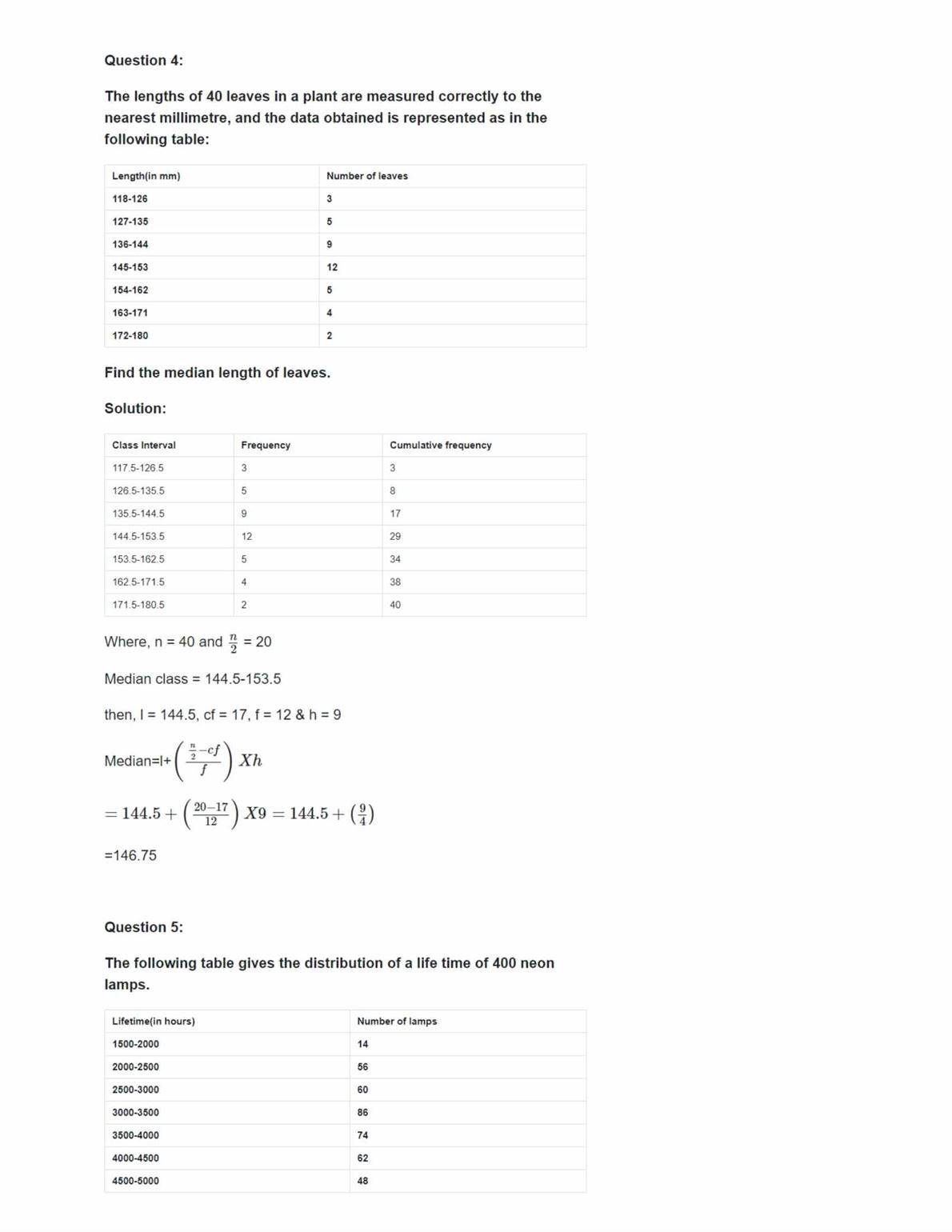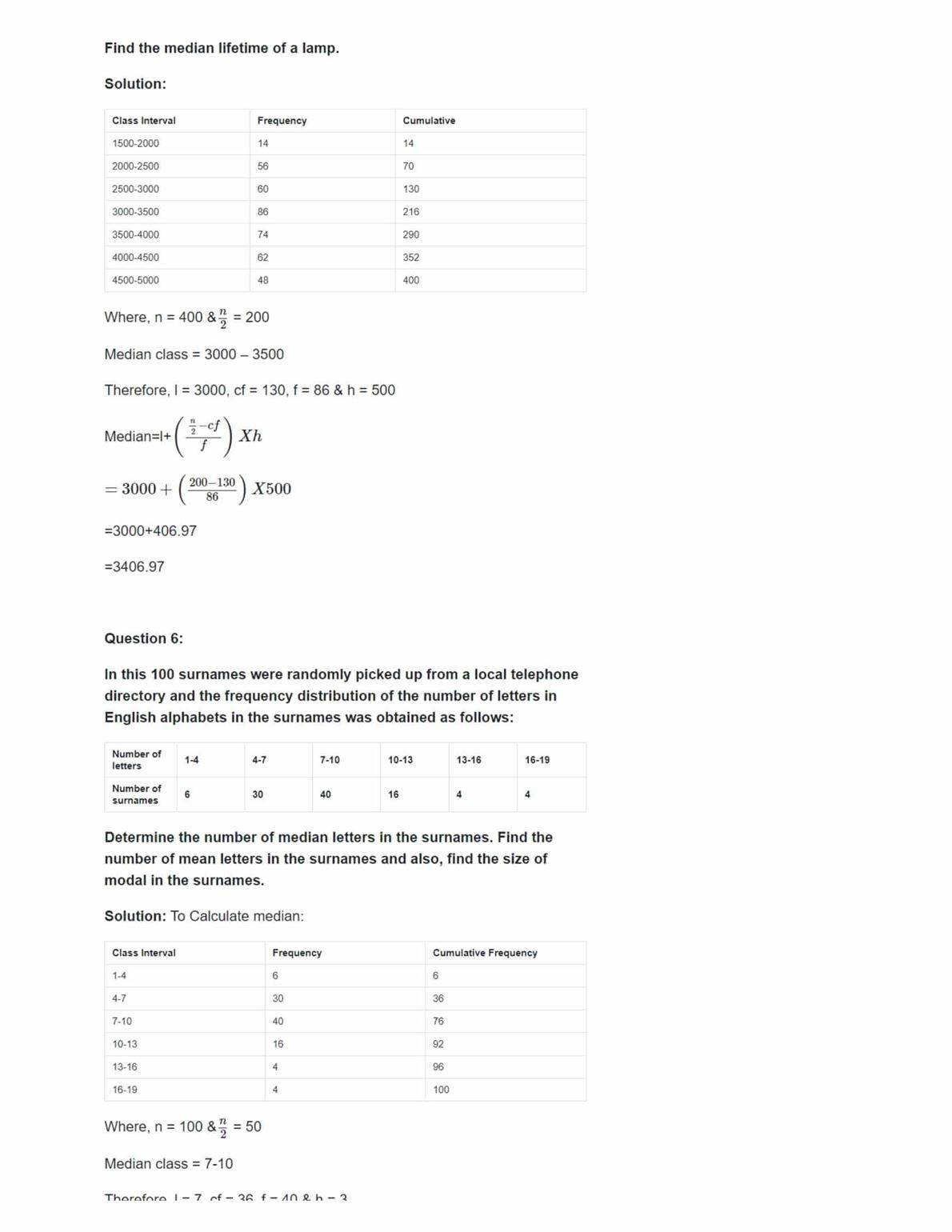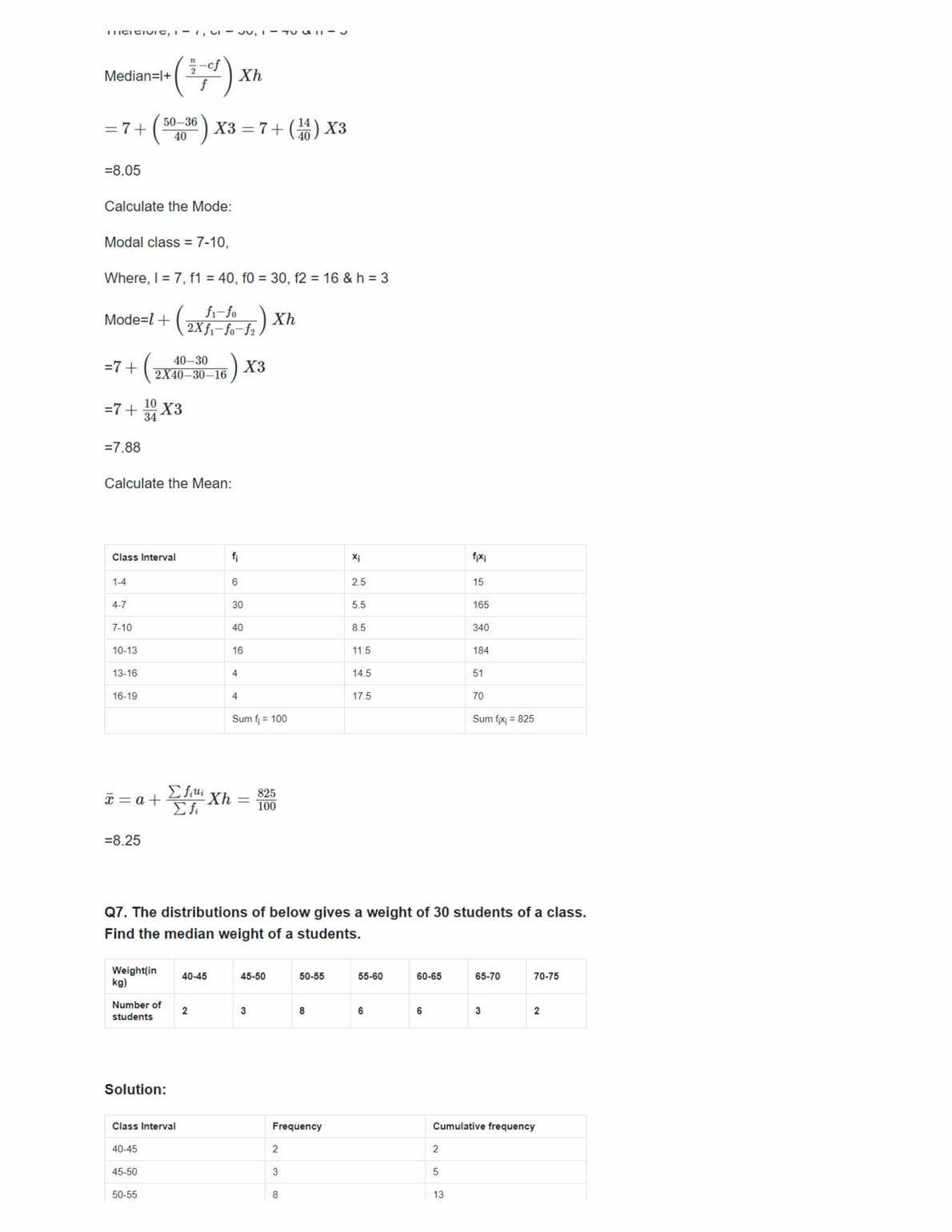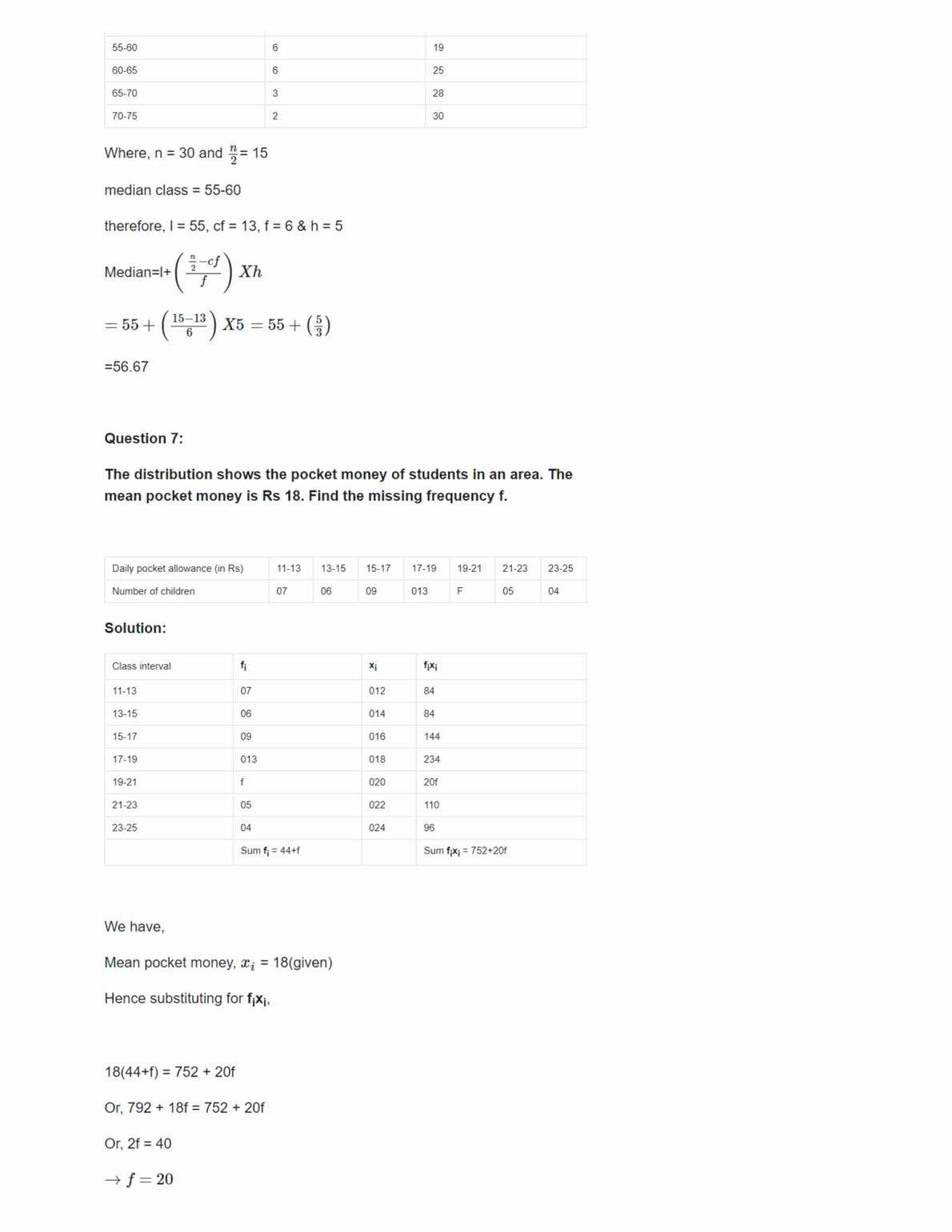Get here NCERT Solutions Class 10 Maths Chapter 14. These NCERT Solutions for Class 10 of Maths subject includes detailed answers of all the questions in Chapter 14 – Statistics provided in NCERT Book which is prescribed for class 10 in schools.

Resource: National Council of Educational Research and Training (NCERT) Solutions
Class: 10th Class
Subject: Maths
Chapter: Chapter 14 – Statistics

## NCERT Solutions Class 10 Maths Chapter 14 – Statistics

NCERT Solutions for Class 10 Maths subject, Chapter 14- Statistics, are provided here, which can be downloaded for free, in PDF format. The Solutions for chapter Statistics are prepared by our experts who have done specialization in Maths.

All the solved questions of Statistics are with respect NCERT syllabus and guidelines, to help students solve each exercise questions, and prepare for the CBSE Board exam. Using this material as a reference tool, will be helpful for them to score good marks. Students can also get here solutions for Class 10th Maths all chapters,exercise-wise.

Chapter 14, Statistics, of Grade 10, is one of the most important of all the chapter present in the textbook. The weightage of this chapter in the final exam is around 11 to 12 marks. On average, there will be 3 questions which could be asked from this chapter and marks will be distributed in a manner of 3+4+4( it could vary as per question).

Topics covered in Chapter 14, Statistics are;

• Mean of Grouped Data
• Mode of Grouped Data
• Median of Grouped Data
• Graphical Representation of Cumulative Frequency Distribution

List of Exercise with a hyperlink

Exercise 14.1 Solutions 9 Question ( 9 long)

Exercise 14.2 Solutions 6 Question ( 6 long)

Exercise 14.3 Solutions 7 Question ( 7 long)

Exercise 14.4 Solutions 3 Question ( 3 long)

NCERT solutions for class 10 maths chapter 14- Statistics are made available for students who want to obtain good marks in this chapter. The methods and procedure to solve the questions have been explained in a broad way, such that, students find it easy to understand the fundamentals, quickly. Students can solutions

The world is highly data-oriented, in fact, each and every field have a group of data which represents the information. Statistics is the branch of mathematics which deals with the representation of data in a meaningful way.

You will face many real-life scenarios where the fundamentals of statistics are used to represent a set of data in tabular form or in graphs or in pie charts. There are a number of methods you will learn from this chapter such as, step deviation methods, finding mode and median of grouped data, converting frequency distribution and the relation between, mode, mean and median methods, etc. 10th Class NCERT solutions are the best material to prepare for the board exam.

• The solutions for the statistics chapter works as a reference for the students.
• It will help students to score marks against the questions asked from the statistics chapter.
• Students can prepare and do the revision for chapter 14 with this source.
• The questions of statistics are solved by subject experts.
• The content of the material is as per the CBSE syllabus and guidelines

### NCERT Solutions For Class 10 Maths Ex 14.1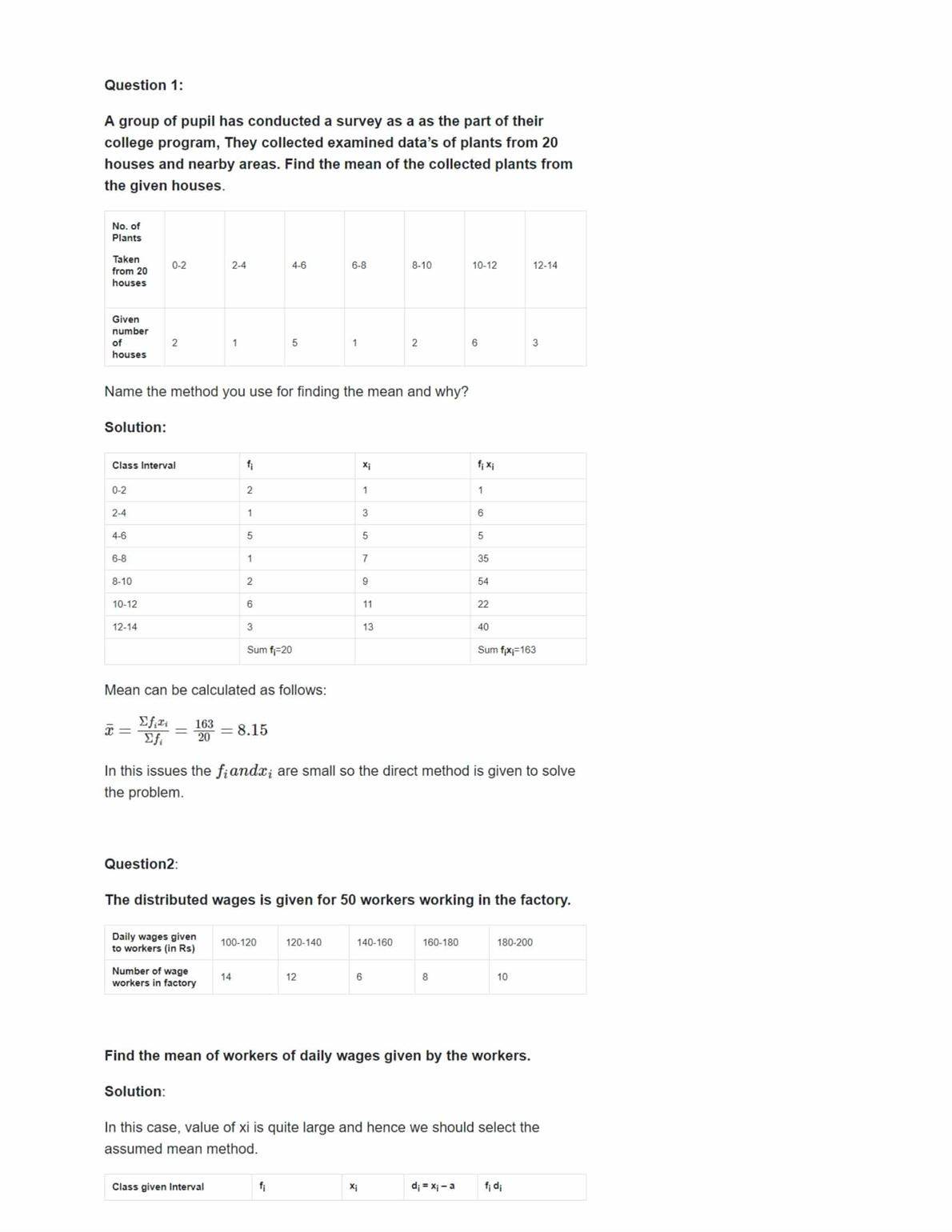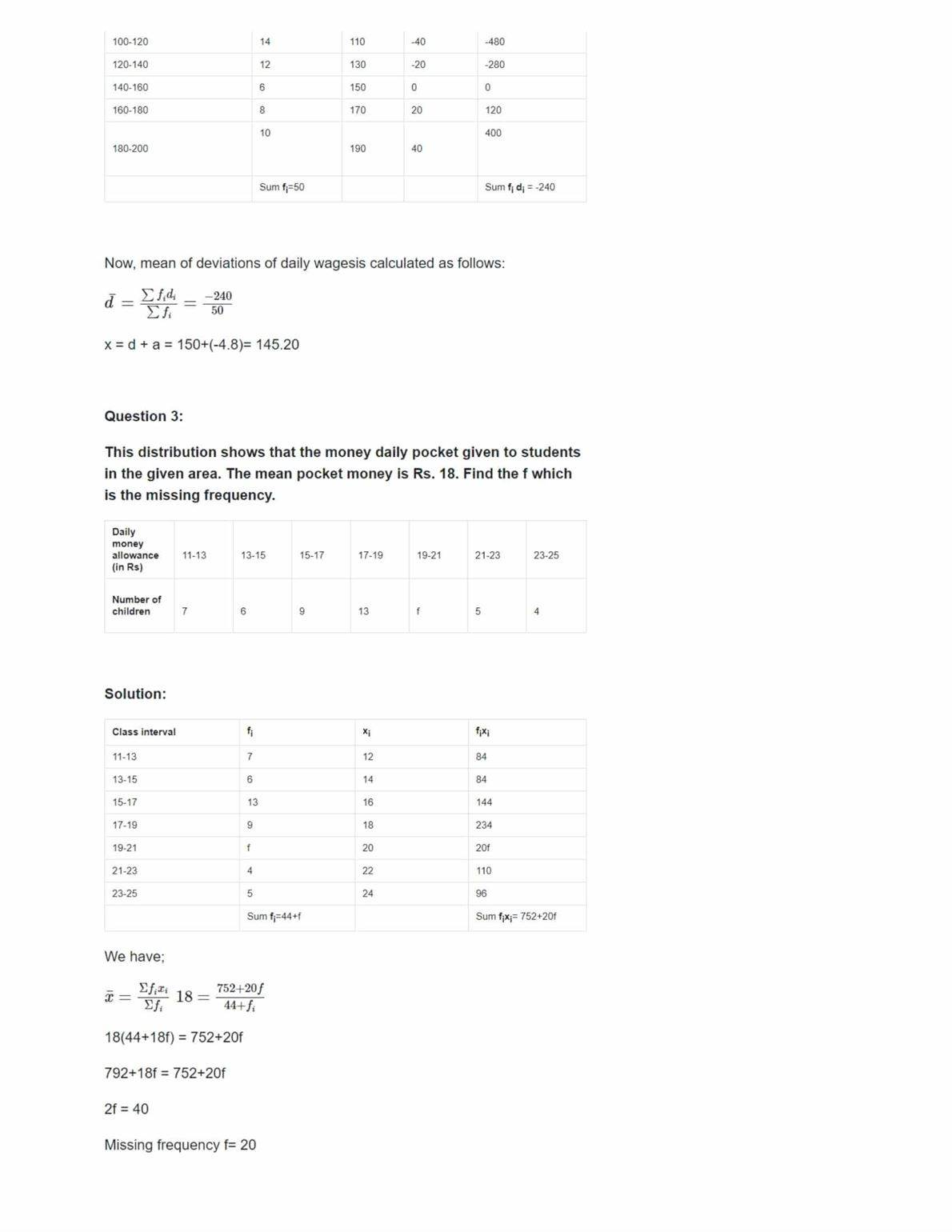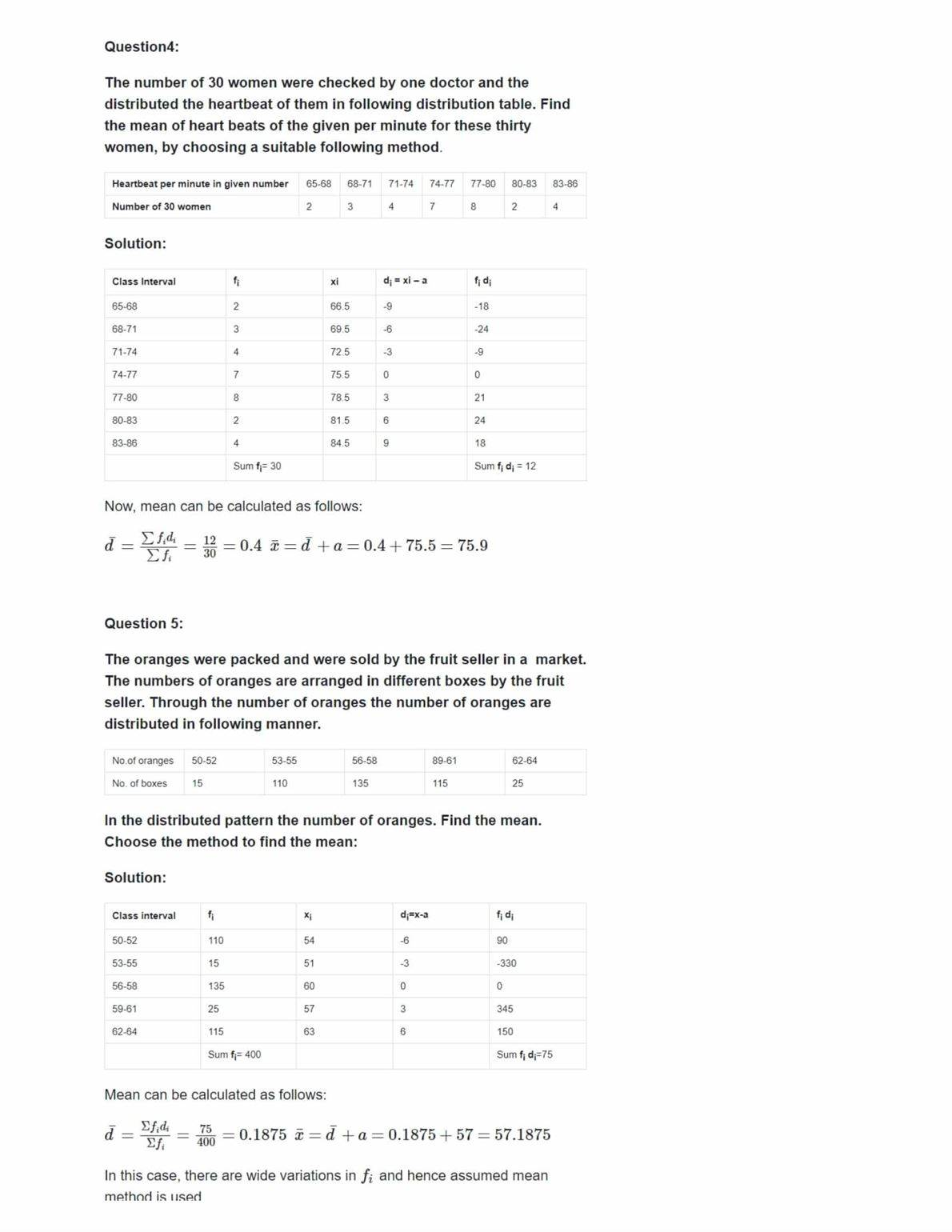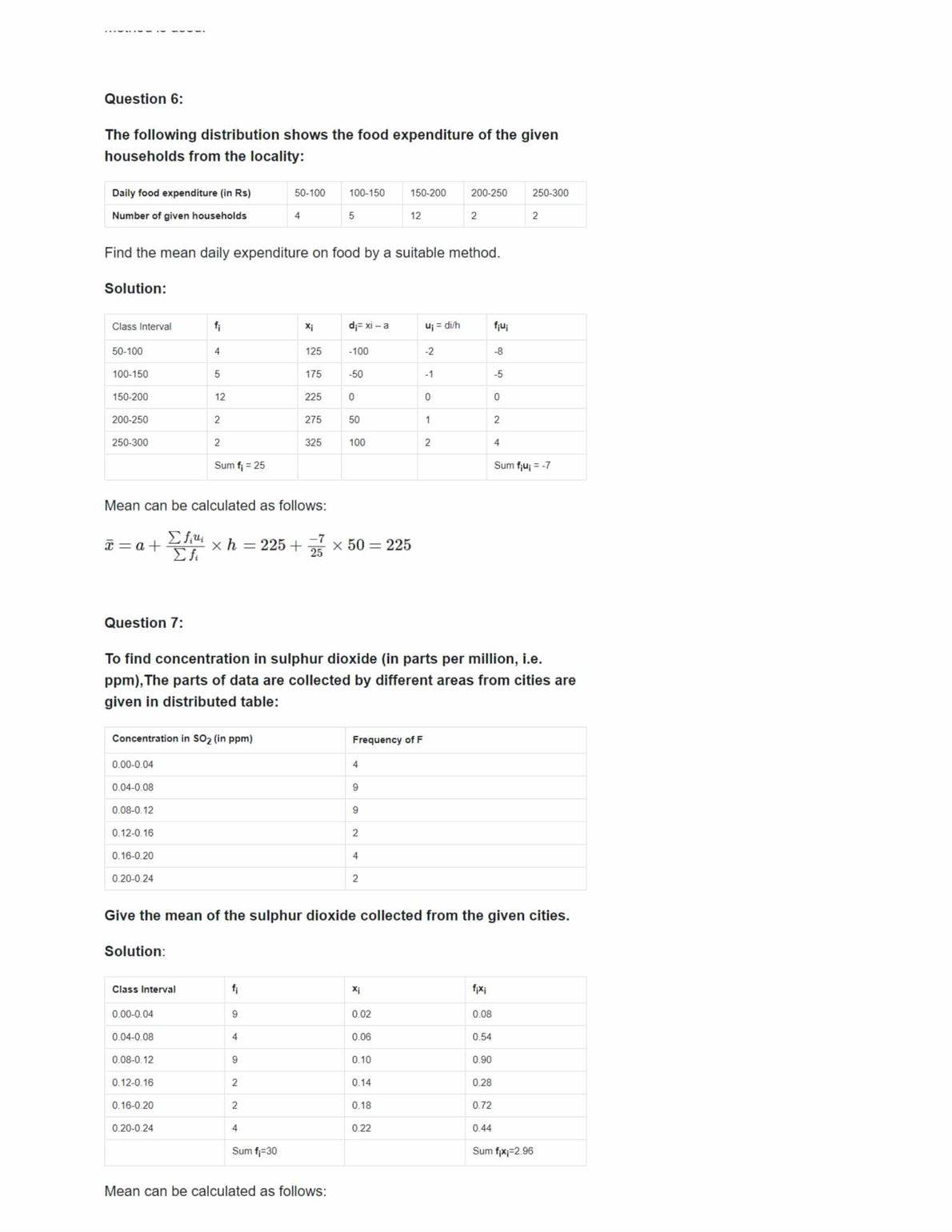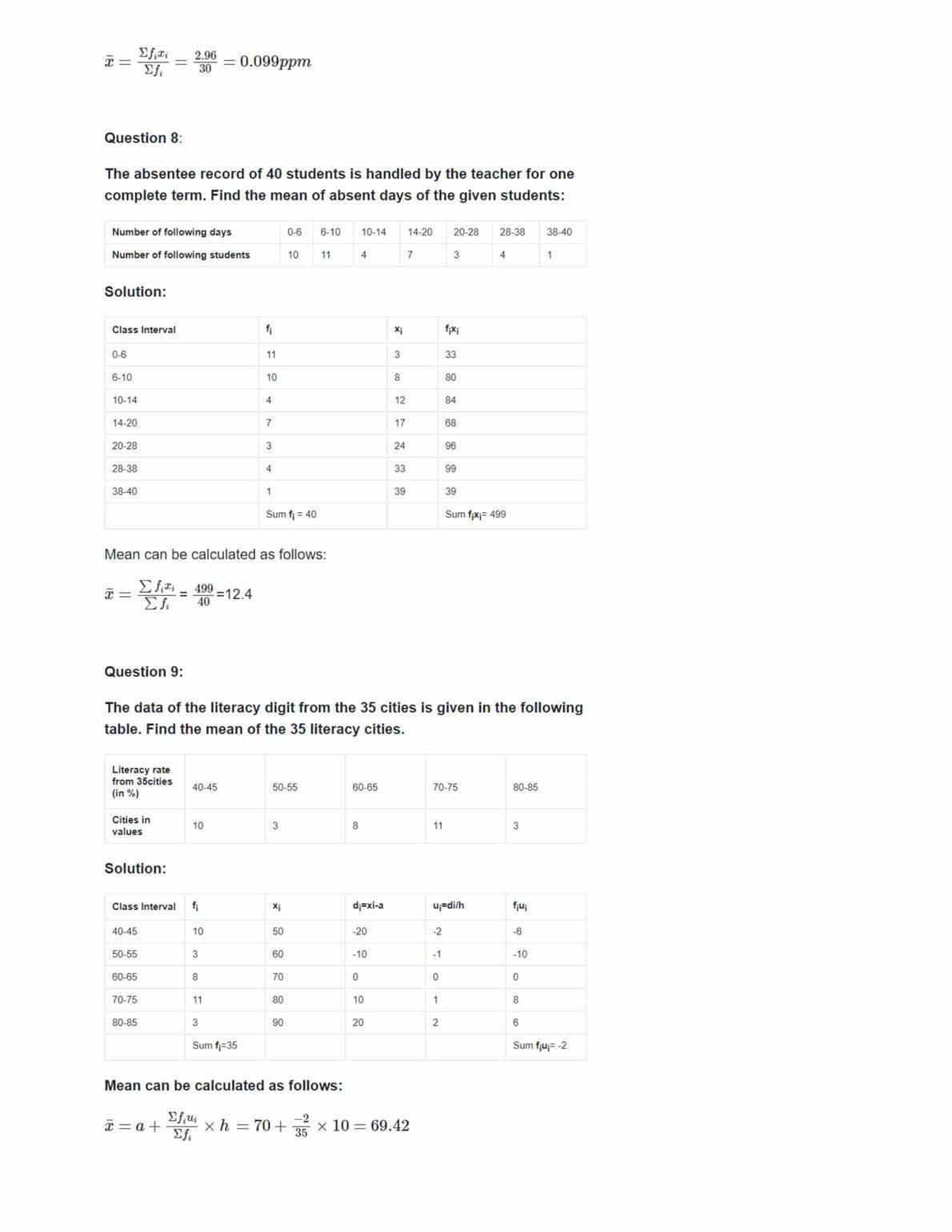### NCERT Solutions For Class 10 Maths Ex 14.2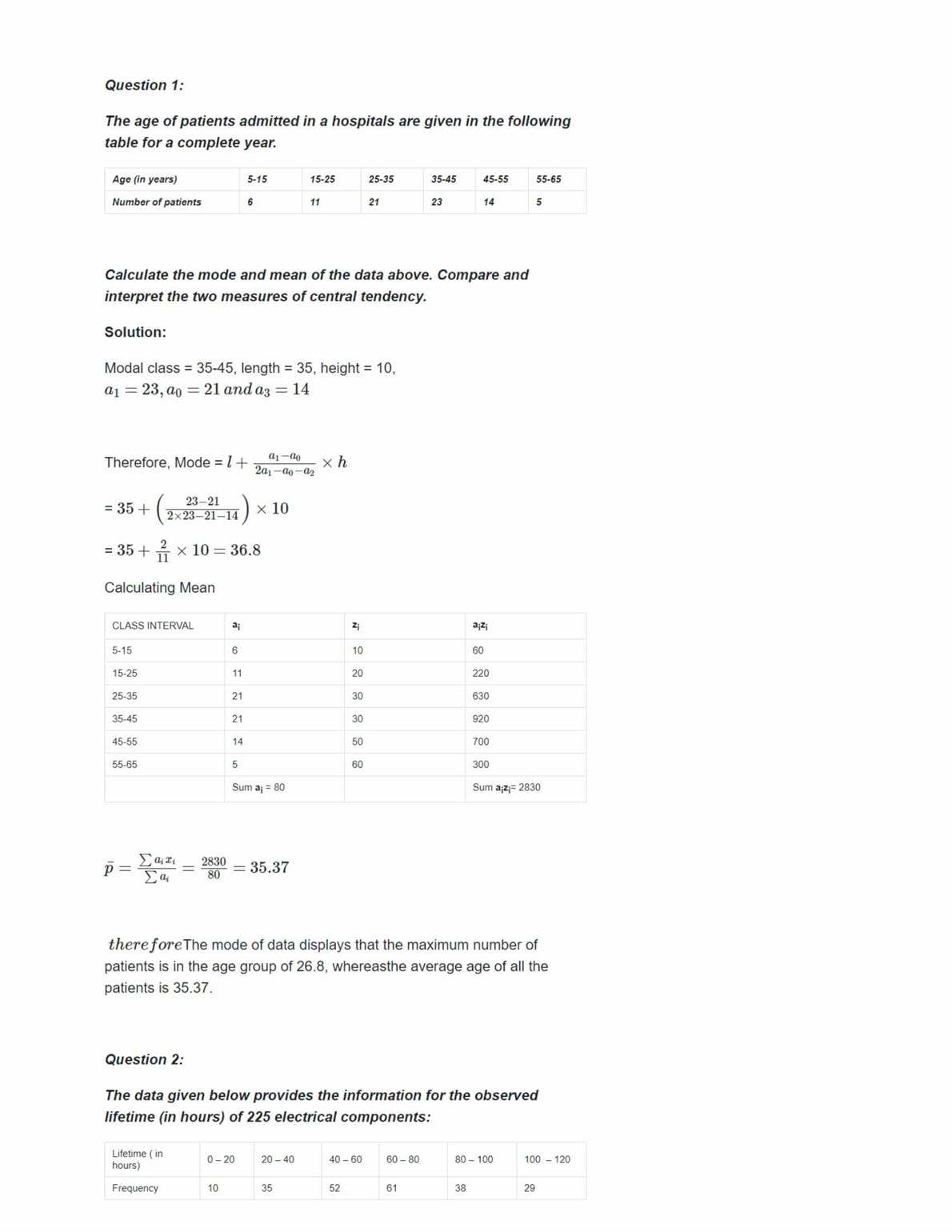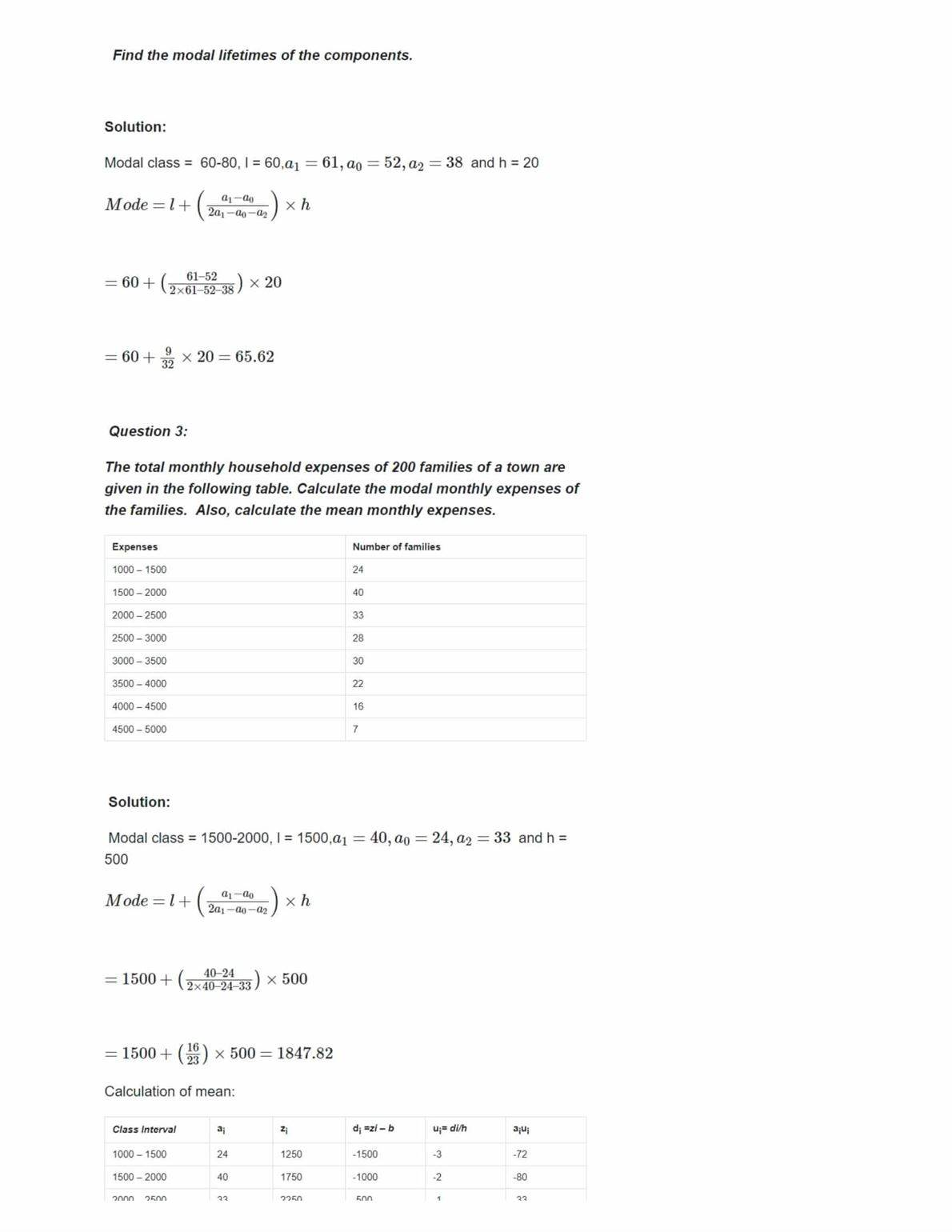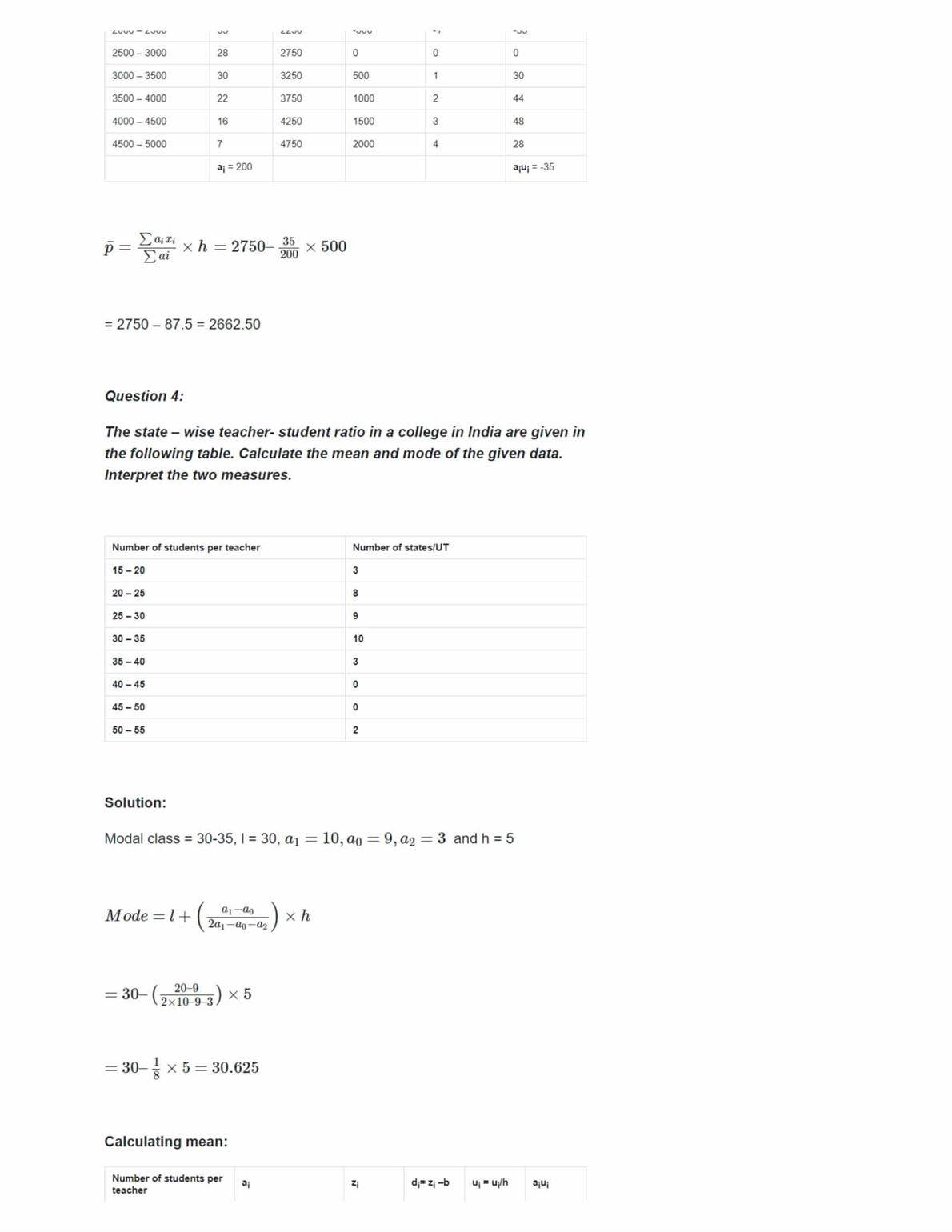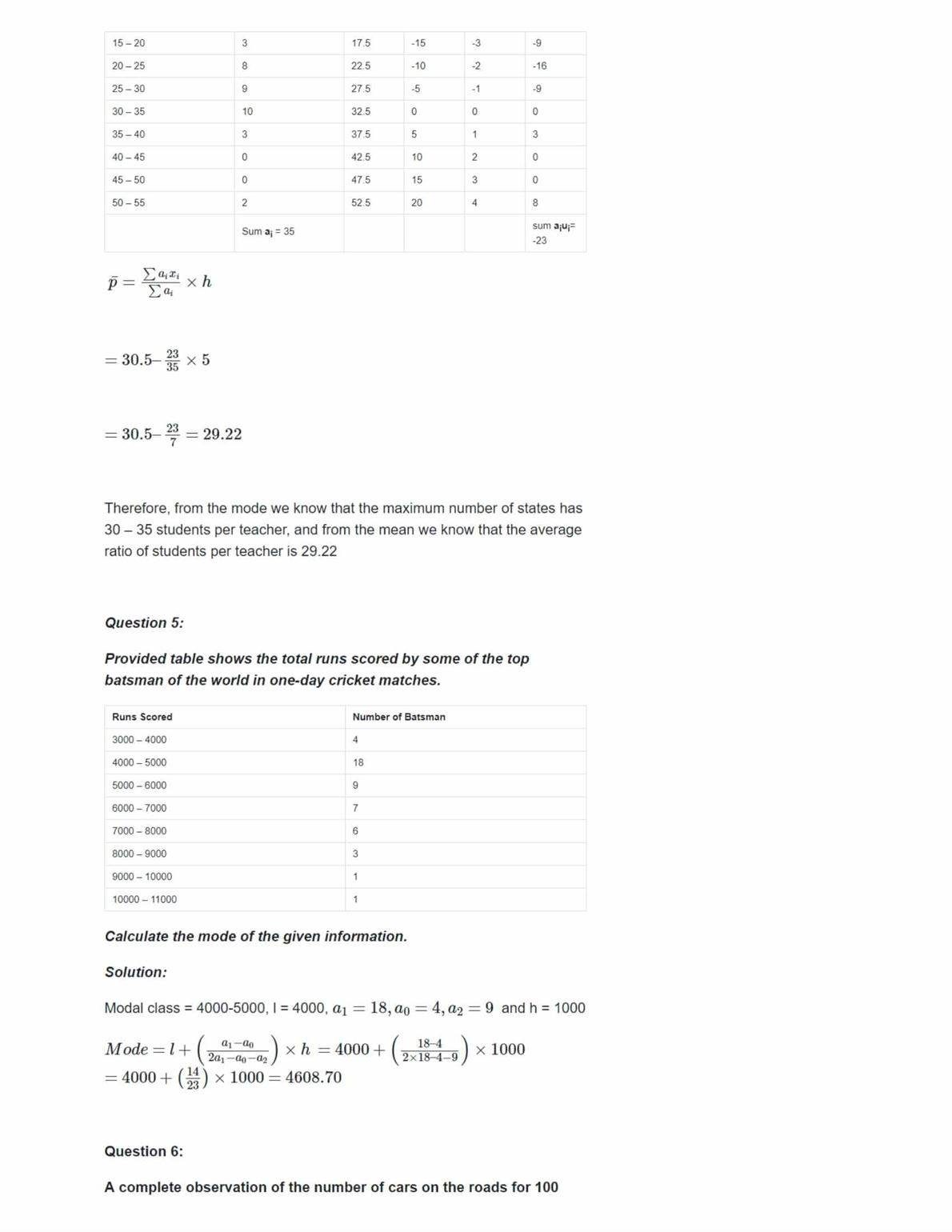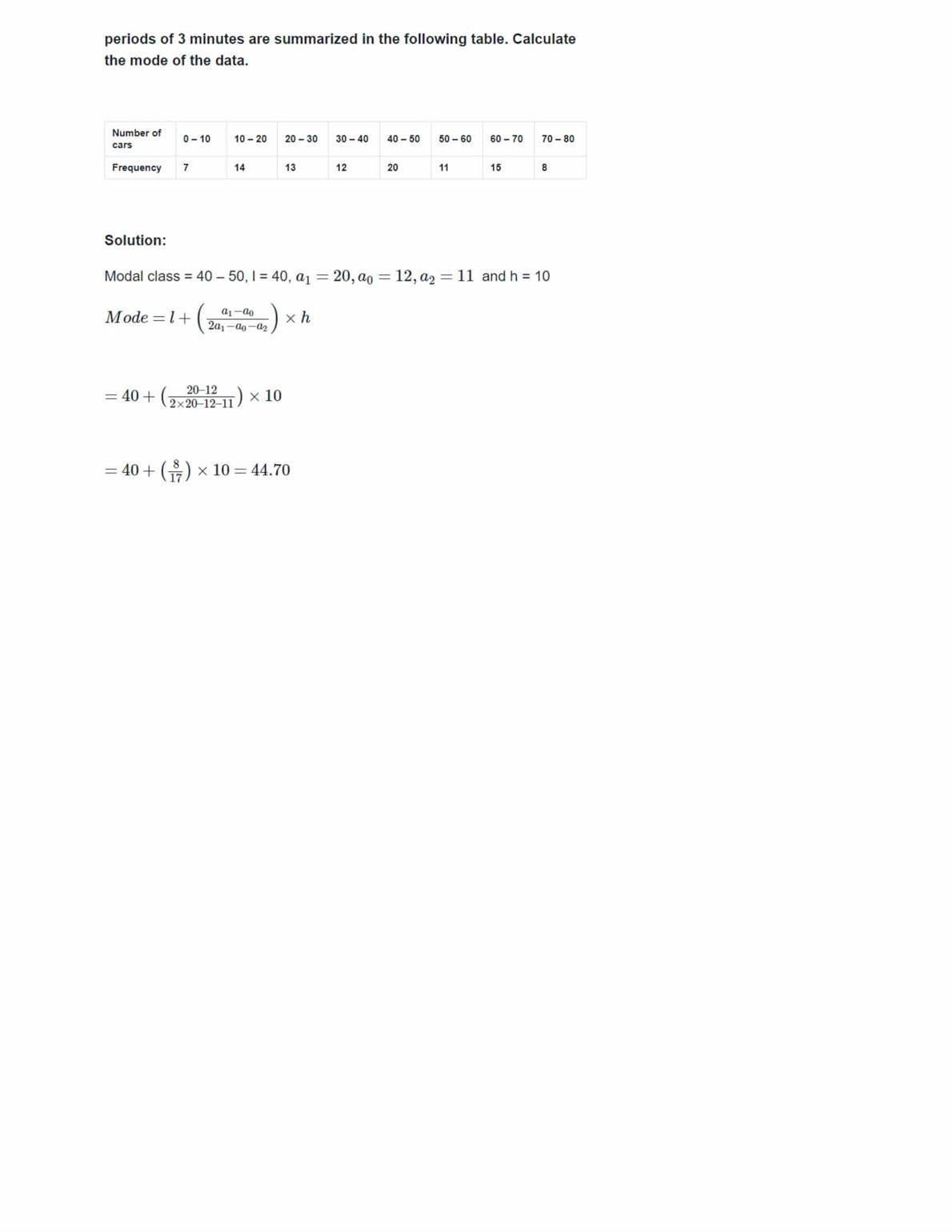### NCERT Solutions For Class 10 Maths Ex 14.3